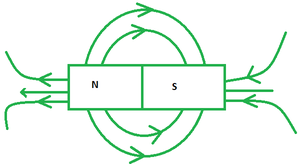Open In App

# Magnetism Formula

Magnetism is a scientific process that is induced by an electromagnetic field. Magnetic fields are generated by the magnetic moments and electric currents of elementary particles. Magnetic fields have a strong attraction for ferromagnetic materials. As a result, they can be easily attracted towards permanent magnets. Cobalt, nickel, iron, and their alloys are examples of ferromagnetic compounds. Temperature, as well as other factors such as magnetic field and pressure, influence a material’s magnetic state.

What is Magnetic Field?

A magnetic field is formed around a wire when an electric current is passed through it. This magnetic field also creates concentric rings around the wire. Furthermore, the magnetic field’s direction is determined by the current’s direction.Furthermore, we may use the ‘right-hand rule,’ which involves pointing the right-hand thumb towards the direction of the current flow. Furthermore, the magnetic field aligns up with the orientation of your folded fingers. The magnitude of the field is determined by the quantity of current and distance from the wire.

Formula

The magnetic effect of an electric field is the property that produces an electric field around the conductor. Prof. H.C Oersted established this phenomenon for the first time in 1820. When he maintained the magnetic needle adjacent to a current-carrying wire, he saw deflection. Ampere’s law indicates the direction in which the needle deflects. The magnetic field’s strength is given by the following formula:

B =where,

• μ0 depicts the allowance of free space (μ0 ​= 4π×10−7T.m/A).
• I refers to the magnitude of current in amperes.
• d and l refer to distance and wire’s length respectively.

### Sample Questions

Question 1. Does the emf in a wire in a magnetic field depend upon the resistance of the loop? If not, why?

Solution:

Since, emf =Here, ϕ = B x A

As a result, the resistance of the loop does not concern the magnitude of the emf produced. Only the area or size of the loop influences it.

Question 2. The strength of a uniform magnetic field through a 2 m long wire with 7 A current is 3.5 T.  Find the force on the wire if it makes an angle of 30 degrees.

Solution:

We know, F = ILBsinθ

Here, I = 7 A, L = 2 m, B = 3.5 T, θ = 30°

⇒ F = (7 A)(2 m)(3.5 T)(sin30°)

⇒ F = 24.5 kg m/s2

Question 3. Consider a loop of wire whose surface is tangential to your vantage point. A constant current flows through the loop in a clockwise direction. What would happen if a magnetic field pointing in your direction were activated?

Solution:

This can be answered using the concept of induction. The current flowing through the loop will adjust to counteract a change in magnetic field. Initially, the loop’s magnetic field is pointing in a direction away from the viewer. As a result, if the external magnetic field is unexpectedly enabled in the direction opposite (towards the spectator), the current in the circuit will reverse this change and boost while staying right to left.

Question 4. While a consistent magnetic flux points into the page, an electron moves at a constant speed to the right somewhere along the plane of the page. Find the direction of the force on it.

Solution:

This question necessitates the use of the right-hand thumb rule. Point your right hand’s fingers in the position of the electron’s velocity (to the right). Turn your thumb in the magnetic field’s direction (into the page). Your palm ought to be approaching the force acting on the electron. However, because electrons are negative, this direction must be overturned, implying that the force is directed upwards through the plane of the page.

Question 5. The strength of a uniform magnetic field through a 0.5 m long wire with 3 A current is 8 T.  Find the force on the wire if it makes an angle of 30 degrees.

Solution:

We know, F = ILBsinθ

Here, I = 4 A, L = 0.5 m, B = 8 T, θ = 30°

⇒ F = (4 A)(0.5 m)(8 T)(sin30°)

⇒ F = 8 kg m/s2